# FORGE Course

## 1. Introduction to Multi-carrier modulation and OFDM

In this chapter we introduce multi-carrier modulation and Orthogonal Frequency-Division Multiplexing (OFDM). We then experiment with real radio hardware equipment on a Future Internet Research & Experimentation (FIRE) testbed facility. Through this course you will gain an appreciation for the factors that are most important in the use of OFDM for wireless communication by exploring its configuration and use on real radios. This will be achieved by completing several well thought out learning exercises, which will reinforce the theory material covered briefly in this chapter and that you have learned previously.

## 2. Learning Outcomes

This chapter will help students become familiar with OFDM concepts.
• Understand the need for OFDM signalling in telecommunications networks.
• Comprehend and understand some of the theory behind multi-carrier modulation and the design of OFDM.
• Observe and examine OFDM performance and behaviour under different conditions using real radio experimentation equipment.
• Exposure to advanced Future Internet Research and Experimentation (FIRE) testbed infrastructure.
• Experience using GNU Radio, an open-source software toolkit that provides signal-processing blocks to implement software radios.

## 3. Multi-carrier Systems and OFDM Standards

The Collins Kineplex System was the first multicarrier system based on orthogonal subcarriers in HF military radio links. It was built in 1957. Several years later in 1966, a team at Bell labs filed a patent (granted in 1979), and published the first article on OFDM systems in IEEE Trans. Communications Technology in 1967 entitled: A proposal of OFDM concepts (Saltzberg, 1967).

OFDM was quickly recognized as an efficient data transmission method and research and standards continued to evolve in the 1980’s, 1990’s and 2000’s with the additional of (see Figure 1):
•     Power-Line-Communication
•     Digital Subscriber Line (DSL) technologies,
•     Broadcast: DAB, DVB-T/-T2, DVB-H, ISDB-T
•     Wireless Personal Area Network (WPAN): WiMEdia
•     Wireless Local Area Network (WLAN): IEEE802.11a/g/n/ac/ad, IEEE 802.15.4g, HiperLAN/2
•     Wireless Metropolitan Area Network (WMAN): IEEE 802.16a WiMAX
•     Mobile telephony: LTE (3.9G), LTE Advanced (4G)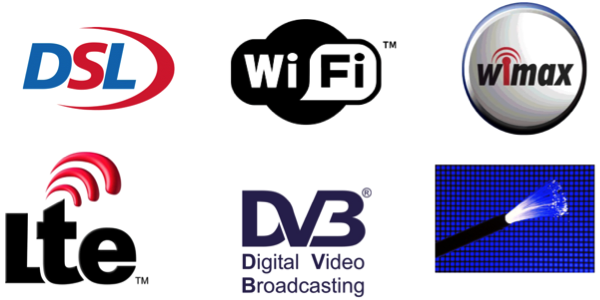Figure 1: OFDM Standards

“OFDM will certainly remain as the root framework for the new 5G waveform design in both the downlink and the uplink, with some optimization to support the new 5G use cases” - Alan Carlton in NetworkWorld, March 2016.

## 4. The Wireless Channel

A signal undergoes changes when it is transmitted on its way to the receiver, see Figure 2. A key metric of the joint impact of a wireless channel is the variation and attenuation in received signal envelope power over time and/or space, which is called fading. There are two types of fading used to describe the signal level at the receiver, large scale-fading and small-scale fading.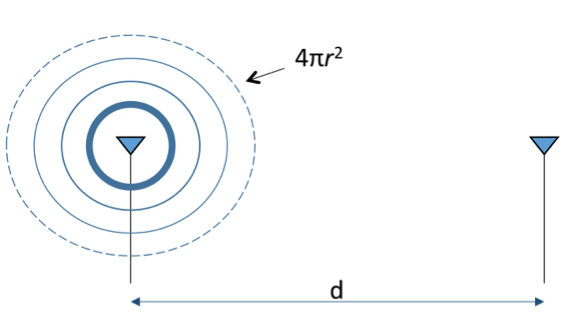Figure 2: Signal Transmitter and Receiver

In large-scale fading, signal power falls quadratically with distance as a result of attenuation and diffraction, which occurs due to the signal traveling over large distances and using different frequencies i.e. signal path loss. Large objects such as trees, buildings, mountains, and so forth cause shadowing, and as a result received power can vary dramatically.

In small-scale fading, which is due to reflectors, scattering and receiver motion, multiple versions of the transmitted signal can be received from different path lengths spread over time. There are several types of small-scale fading. These include: Multipath and Motion.

#### 4.2.1 Multipath

If the channel is considered as a linear-time-invariant system, the convolution of the channel impulse response h(t,τ) with the input stimulus x(t) (the transmitted signal) yields the system output y(t) (the channel output, i.e. the received signal). Delay spread σ↓τ is the maximum difference between times of arrival among multipath components. The following video illustrates multipath small-scale fading:

#### 4.2.2 Motion

If transmitter, receiver and/or interacting objects are in motion with the speed v under relative angle ϕ, the received signal gets shifted in frequency by Δf due to the Doppler effect, i.e., Doppler shift – see Figure 3.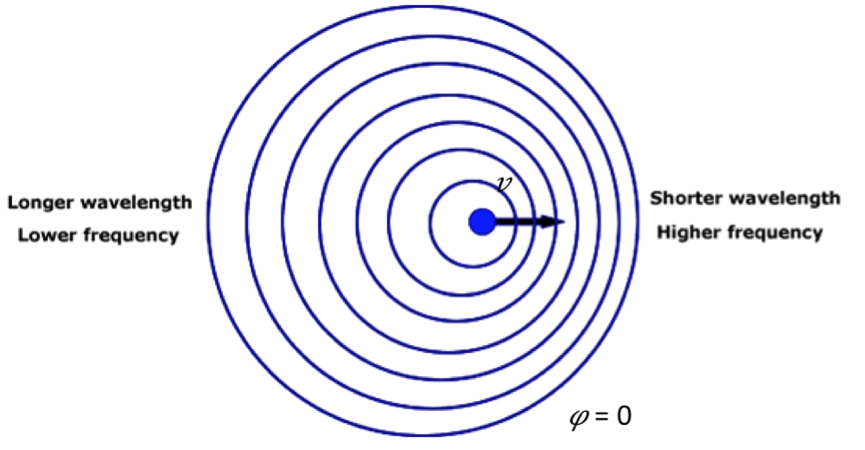Figure 3: The Doppler Shift

Different propagation directions result in different Doppler shifts per multipath component. Received envelope power depends on constructive or destructive addition of signals. The following short video gives a good explanation of the Doppler Effect:

## 5. Multi-carrier Systems

Multimedia applications require higher and higher data rates from wireless and wired communications systems. Mobile radio channels are fading channels that can be flat or frequency selective. For high bandwidth applications channels are frequency selective. In conventional single-carrier modulation techniques this can only be achieved by, see Figure 4:
Transmitting shorter symbols => limited in case of multi-path propagation (Inter-symbol interference (ISI))
Transmitting more bits per symbol => limited by noise and other distortions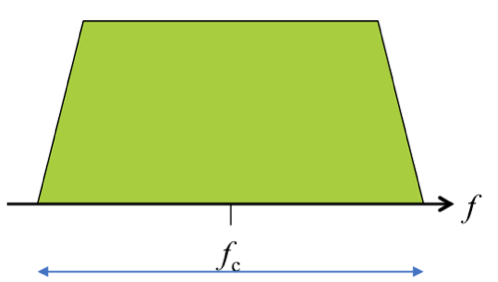Figure 4: Single carrier/mono-carrier system

Symbol width: 1/W and data is transmitted over only one carrier. Disadvantages include:
•     Equalization is complex
•     Very short pulses
•     Inter-symbol interference (ISI) is long
•     Poor spectral efficiency because of guard bands

Multicarrier modulation is a technique where multiple low data rate carriers are combined by a transmitter to form a composite high data rate transmission, see Figure 5. To improve the spectral efficiency, guard bands between carriers need to be eliminated. In a classic multi-carrier system, the available spectrum is split into several non-overlapping frequency sub channels. The individual data elements are modulated into these sub channels and are thus frequency multiplexed.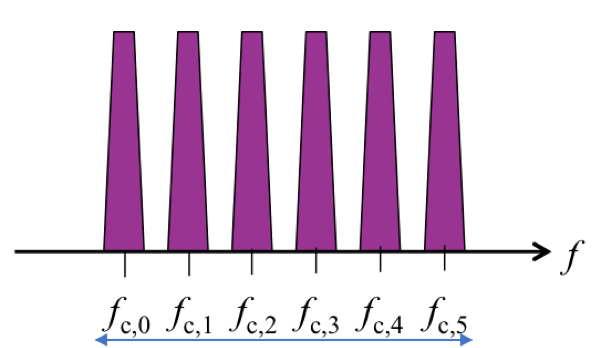Figure 5: Multicarrier system

Symbol width= N_c/W and data stream is split up into multiple lower-data rate sub streams, see Figure 5. They are modulated and transmitted in parallel on different sub carrier frequencies i.e. Frequency Division Multiplexing (FDM). By parallel data transmission on NC sub-carriers, symbol duration TS can be increased by factor N_c  to achieve the same data rate. Longer symbols are less susceptible against inter-symbol interference (ISI). Other advantages include:
•     N_c short equalizers
•     N_c long pulses
•     ISI is relatively short
•     Poor spectral efficiency because of guard bands
•     It is easy to exploit Frequency diversity
•     2D coding techniques are allowed
•     Dynamic signalling is possible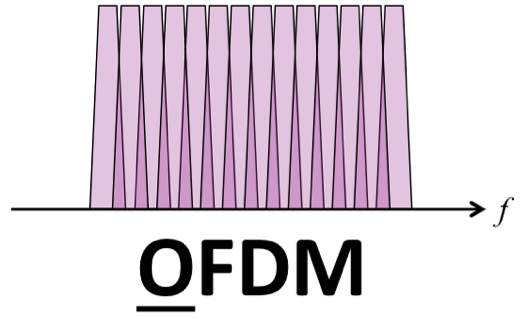Figure 6: OFDM subcarrier tones are separated by the inverse of the signalling symbol duration

## 6. Orthogonal Frequency-Division Multiplexing (OFDM)

Orthogonal frequency-division multiplexing (OFDM) is a multicarrier modulation technique for encoding digital data on multiple carrier frequencies. It is an FDM scheme that uses a large number of sub-carrier signals. These signals are orthogonal to each and carry parallel channels of data. In classic multicarrier systems, guard bands have to be inserted, resulting in poor spectral efficiency. A more efficient approach is to allow the spectra of individual subcarriers to overlap, see Figure 6. Zero crossings occur at every multiple of and hence no inter-carrier interference is present i.e., no overlap at sampling frequencies. The following YouTube video gives a high-level overview of OFDM technology:

Problem: If individual subcarriers are overlapping isn’t there interference between carriers?

Answer: No!  If subcarrier tones are separated by the inverse of the signalling symbol duration, independent separation of frequency-multiplexed tones is possible. Additionally, sub-spectra may overlap in frequency domain, which supports more efficient use of available spectrum and greater data rates are achievable.

In the remainder of this section we give you a brief overview of some basic OFDM concepts that we will explore further in the experimentation section using TCDs IRIS FIRE testbed equipment. These include, symbol mapping/de-mapping, Inverse Discrete Fourier Transform (IDFT), Discrete Fourier Transform (DFT), equalization, cyclic prefix, and frequency sensitivity. Figure 7 illustrates the OFDM Systems Model, and how these concepts are interconnected.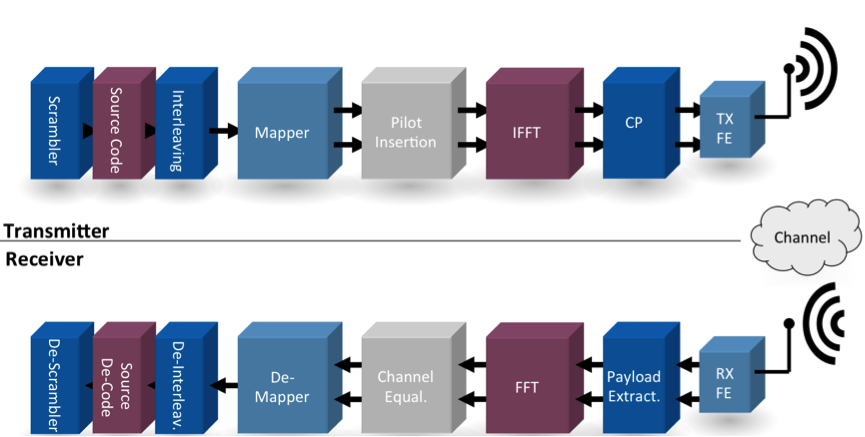Figure 7: The OFDM System Model

## 6.1 Symbol Mapping / De-Mapping

Symbol mapping (or de-mapping) involves loading (or unloading) data bits received from the source encoder and the interleaver (or channel equalizer) to (or from) complex subcarrier modulations such as QAM, PSK, and so forth, see Figure 7.

### 6.2 Inverse Discrete Fourier Transform (IDFT)

The output from the mapper constitutes as input to the Inverse Discrete Fourier Transform (IFFT), which accepts complex input data. In IDFT, data is parallelized then treated as samples in the frequency domain. The IDFT process transforms these into time domain signals. Rectangular time-domain pulse shaping spectra of the subcarriers becomes a cardinal sine function or sinc function in the frequency domain.

If number of sub-carriers NC is chosen as a power of 2 (2, 4, 8, 16, 32, 64, 128, 256, 512, 1024, 2048), the IDFT can be replaced by an IFFT, yielding a very efficient implementation of a OFDM modulator (FFT for demodulator at receiver). For example, 8-PSK, which has 8 Phase Shift Keying, has three bits per sub-carrier per symbol, see Figure 8.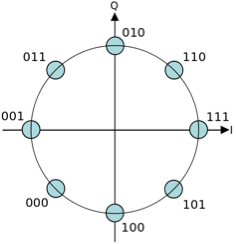Figure 8: 8-psk Constellation Diagram

### 6.3 Equalization

The primary advantage of OFDM over mono-carrier schemes is its ability to cope with severe attenuation across channel frequencies such as small or large-scale fading (discussed above in Section 4) using a simplified equalisation scheme. Equalisation helps attenuate or adjust the balance between frequency components to flatten channel response, supporting the removal of frequency selective fading effects. This is achieved by:
•     Insertion of known symbols (pilots) in the OFDM frame
•     Evaluating their distortions at the receiver
•     Assuming a relatively static channel, data symbols can be equalized

In OFDM, each carrier becomes a infinite sinusoid (i.e. eigenfunction). As a result, the out of channel is a scaled version of the same function. The eigenvalues of the (circular) channel are the complex scalar terms that multiply each carrier. Thus symbols only experience magnitude and phase change, which makes equalization simple. Convolution in time domain corresponds to multiplication in frequency domain. However, this fact does not hold in discrete time. Circular convolution in (discrete) time domain corresponds to multiplication in (discrete) frequency domain. OFDM wants simple multiplication in the frequency domain. So, circular convolution is needed and not the regular convolution i.e., real channel does regular convolution. The solution to this problem is to add a cyclic prefix, so regular convolution can be used to create circular convolution.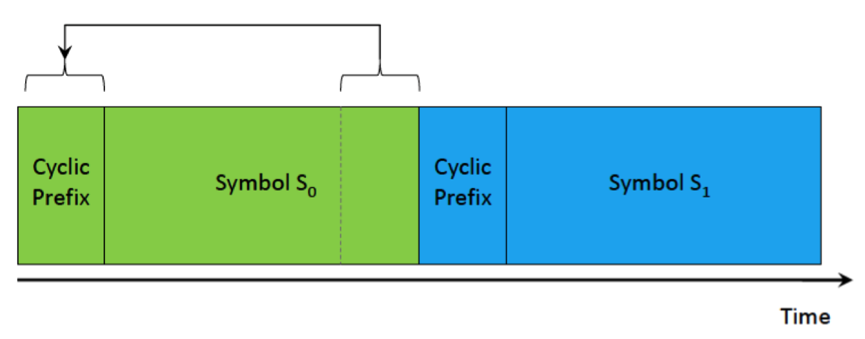Figure 9: Cyclic prefix

### 6.4 Cyclic Prefix

The cyclic prefix is added to beginning of a symbol and is a repetition of the end of a symbol. Figure 9 shows the cyclic prefix added to a symbol over time. Its purpose is to help preserve sinusoids in multipath channels. Sinusoids are eigenfunctions of linear time-invariant channels. The cyclic prefix helps eliminate inter-symbol interference (ISI), which is the delayed replica of previous symbols interfering with the current symbol. Additionally, they facilitate equalization by transforming linear convolution into circular convolution. Transmission time is limited to N symbols and this property is lost. The cyclic prefix restores this property by “simulating” an infinite-length sinusoid. Looking at the spectrum, Y(f)=X(f)·H(f), if H(f) is not approximately equal for all f, the original signal is destroyed. Cyclic prefix can make OFDM transmissions completely immune to ISI created by multipath propagation when cyclic prefix length T_cp is longer than the delay spread:
T_cp ≥ σ↓τ

### 6.5 Discrete Fourier Transform (DFT)

At the receiver, OFDM de-modulation uses Discrete Fourier Transform (DFT) transformation to convert payload received to the frequency domain. Modulation symbols received from the DFT are de-mapped from complex subcarrier modulations such as QAM, PSK, and so forth, to bits, which are inputted into the deinterleaver and the source-decoder blocks.

## 7. OFDM Disadvantages: Timing and Frequency Sensitivity

OFDM transmissions are susceptible to timing and frequency offsets. Timing offsets are due to uncertainties of OFDM symbol boundaries, which can cause intersymbol interference, channel interference and phase offset. Frequency offsets cause inter-carrier interferences (ICI), and a reduction of desired power in data received. Frequency offsets are caused by the Doppler shift or hardware imperfections e.g. imprecise up-/down-conversion. This has the effect that operating on different frequency sub-carriers is no longer orthogonal. OFDM needs accurate frequency synchronisation. This is explored further in experimentation question 10.3 below.

## 8. OFDM Data Rates

Doubling subcarriers in used bandwidth does not double the data rate. See Table 1 for comparisons of modulation depth and data rate.
 Data Rate Bandwidth N Code Rate Modulation 6 Mbps 15 48 1/2 BPSK 9 Mbps 15 48 3/4 BPSK 12 Mbps 15 48 1/2 QPSK 18 Mbps 15 48 3/4 QPSK 24 Mbps 15 48 1/2 16-QAM 36 Mbps 15 48 3/4 16-QAM 48 Mbps 15 48 2/3 64-QAM 54 Mbps 15 48 3/4 64-QAM
Table 1: OFDM Data Rates and Modulations Depths

## 9. FIRE Testbed Environment

This OFDM course runs completely on Trinity College Dublin’s (TCD’s) IRIS testbed facility, which is located on TCD’s campus in Dublin, Ireland. The testbed consists of 16 flexible Universal Software Radio Peripheral (USRP) N210 Ettus Research units aligned in a grid configuration. Each USRP is connected to a virtual machine that runs a software-defined radio (SDR) system. In these experiments we use the GNU Radio software development toolkit. GNU Radio offers signal-processing blocks that implement software radios. A conceptual diagram of IRIS’s virtualized cloud resources, radio hypervisor, user experiments and physical equipment is shown in Figure 10. The hardware that you will use will be configured automatically through a process called provisioning. This process will take care of the reservation of two virtual machines in TCDs FIRE testbed facility, the connection of the same to appropriate USRP hardware, installation of required operating system and tools, and initialization of experimentation services. These two virtual machines are under your sole control for use in experimentation. Data for monitoring wireless spectrum is sent to a database on the webserver which is displayed to you via a graph.